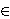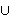Nikica Uglešić and Branko Červar

University of Zadar, Studentski dom, F. Tuđmana 24 D, 23000 Zadar, Croatia
e-mail: nuglesic@unizd.hr

Department of Mathematics, University of Split, Teslina ulica 12/III, 21000 Split, Croatia
e-mail: brankoch@pmfst.hr

Abstract.   By reducing the Mardešić S-equivalence to a finite case, i.e., to each n{0}N separately, we have derived the notions of Sn-equivalence and Sn+1-domination of compacta. The Sn-equivalence for all n coincides with the S-equivalence. Further, the Sn+1-equivalence implies Sn+1-domination, and the Sn+1-domination implies Sn-equivalence. The S0-equivalence is a trivial equivalence relation, i.e., all non empty compacta are mutually S0-equivalent. It is proved that the S1-equivalence is strictly finer than the S0-equivalence, and that the S2-equivalence is strictly finer than the S1-equivalence. Thus, the S-equivalence is strictly finer than the S1-equivalence. Further, the S1-equivalence classifies compacta which are homotopy (shape) equivalent to ANR's up to the homotopy (shape) types. The S2-equivalence class of an FANR coincides with its S-equivalence class as well as with its shape type class. Finally, it is conjectured that, for every n, there exists n' > n such that the Sn'-equivalence is strictly finer than the Sn-equivalence.

2000 Mathematics Subject Classification.   55P55.

Key words and phrases.   Compactum, ANR, shape, S-equivalence.

Full text (PDF) (free access)

DOI: 10.3336/gm.42.1.14

References:

1. K. Borsuk, Some quantitative properties of shapes, Fund. Math. 93 (1976), 197-212.
MathSciNet

2. D. A. Edwards and R. Geoghegan, Shapes of complexes, ends of manifolds, homotopy limits and the Wall obstruction, Ann. of Math. (2) 101 (1975), 521-535, Correction. Ibid. 104 (1976), 389.
MathSciNet     CrossRef

3. K. R. Goodearl and T. B. Rushing, Direct limit groups and the Keesling-Mardesic shape fibration, Pacific J. Math. 86 (1980), 471-476.
MathSciNet

4. H. M. Hastings and A. Heller, Splitting homotopy idempotents, in: Shape theory and geometric topology (Dubrovnik, 1981), Lecture Notes in Math. 870, Springer, Berlin-New York, 1981, 23-36.
MathSciNet

5. J. Keesling and S. Mardesic, A shape fibration with fibers of different shape, Pacific J. Math. 84 (1979), 319-331.
MathSciNet

6. S. Mardesic, Comparing fibres in a shape fibration, Glasnik Mat. Ser. III 13(33) (1978), 317-333.
MathSciNet

7. S. Mardesic and J. Segal, Shape Theory, North-Holland Publishing Co., Amsterdam-New York-Oxford 1982.
MathSciNet

8. S. Mardesic and N. Uglesic, A category whose isomorphisms induce an equivalence relation coarser than shape, Topology Appl. 153 (2005), 448-463.
MathSciNet     CrossRef

9. K. Morita, The Hurewicz and the Whitehead theorems in shape theory, Sci. Rep. Tokyo Kyoiku Daigaku Sect. A 12 (1974), 246-258.
MathSciNet

10. N. Uglesic, A note on the Borsuk quasi-equivalence, submitted.

11. N. Uglesic and B. Cervar, A subshape spectrum for compacta, Glasnik Mat. Ser. III 40(60) (2005), 351-388.
MathSciNet

12. C. T. C. Wall, Finiteness conditions for CW-complexes, Ann. of Math. (2) 81 (1965), 59-69.
MathSciNet     CrossRef